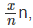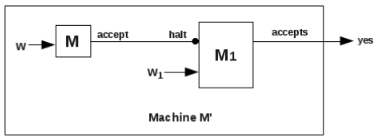Courses

# Church’s Thesis, Godelization, Time Complexity of Turing Machine and Halting Problem of TM Computer Science Engineering (CSE) Notes | EduRev

## Computer Science Engineering (CSE) : Church’s Thesis, Godelization, Time Complexity of Turing Machine and Halting Problem of TM Computer Science Engineering (CSE) Notes | EduRev

The document Church’s Thesis, Godelization, Time Complexity of Turing Machine and Halting Problem of TM Computer Science Engineering (CSE) Notes | EduRev is a part of the Computer Science Engineering (CSE) Course Theory of Computation.
All you need of Computer Science Engineering (CSE) at this link: Computer Science Engineering (CSE)

Part V. Church’s Thesis

8 Church’s Thesis
It is also called Church- Turing thesis.
Church’s thesis states that
A function is said to be computable if it can be computed by a Turing machine.

This thesis talks about mechanical computation devices and the kind of computations they can perform.
Some more definitions of this thesis are given below:
1. Any mechanical computation can be performed by a Turing machine.
2. For every computational problem, there is a corresponding Turing machine.
3. Turing machines can be used to model any mechanical computer.
4. The set of languages that can be decided by a TM is the same as that which can be decided by any mechanical computing machine.
5. If there is no TM that decides problem P, there is no algorithm that can solve problem P.

Part VI. Godelization

9 Godelization
Godelization is an encoding technique which encodes a string as a number. This is called as Godel numbering.
Godel numbering is based on the concept that every positive integer can be factored into a unique set of prime factors.
For example,
6 = 2 x 3
8 = 2 x 2 x 2
9 = 3 x 3
10 = 2 x 5
20 = 2 x 2 x 5
30 = 2 x 3 x 5
50 = 2 x 5 x 5
100 = 2 x 2 x 5 x 5
5,71,725 = 3 x 3 x 3 x 5 x 5 x 7 x 11 x 11
Also, it is possible to assign a serial number to each prime number, as
1 to 2
2 to 3
3 to 5
4 to 7
5 to 11, and so on.
Now the number,
5,71,725 = 3 x 3 x 3 x 5 x 5 x 7 x 11 x 11 = 20 � 33 � 5� 71 � 112
can be represented in the form of a sequence (0, 3, 2, 1, 2).
In terms of Godel numbering, we say that the Godel number associated with the sequence (0, 3, 2, 1, 2) is 5,71,725.

Thus the formal definition of Godel numbering is,
For any finite sequence (x0, x1, x2, .......xn) the associated Godel number, Gn is,
Gn(x0, x1, x2, .......xn) = 2x0 3x1 5x2 7x3.........Pwhere Pn is the nth prime number.

The importance of Godel numbering lies in the fact that each Godel number encodes a unique sequence and a sequence encodes a unique Godel number.
This allows us to represent different configurations of a multi parameter dependent object in the form of a unique number.
A TM at any instant, can exist in different configurations, with each configuration being defined by the current state and position of the head. The position of the head can be defined by a cell number. It is possible to number each state and each cell, and to encode every configuration of a TM in the form of a Godel number. Also, it is possible to define every
transition function of a TM in the form of a Godel number.

Part VII. Time Complexity of Turing Machine

10 Time Complexity of Turing Machine
A Turing machine takes a string w, it gives the output in the form yes or no. Here it is possible to exactly measure the
number of moves made by the head of the TM during the processing of the string.
Although the number of moves made by the TM to process the string depend on the specific string, it is possible to
make an estimate of the maximum possible number of moves that TM will make to accept or reject a string of length of n.
Time complexity of a Turing machine, M is written as,
τM(n)
where τ is the time complexity, n is a natural number.
Time complexity of a TM is the maximum number of moves made by the head to accept or reject a string of length n in
the worst case scenario.

Example 1:
Consider the TM to accept the language, L = {wwR|w ∈ (a, b)+}
Find the time complexity of the TM.
Let the string is of length n.
Here the TM works as follows:
It checks the first symbol of the input string, converts it to #, and travels the whole of the string and reaches the # just
following the string. It turns back and matches the last symbol of the string with the first one. If the match occurs, this last
symbol is replaced with a #. Next the head travels back to the second symbol of the input string.

Here the number of moves required is 2n + 1.
On reaching the second symbol, it finds its corresponding match.
Here the number of moves required is 2n - 3.
When al lthe pairs are successfully matched, TM just makes one move and halts.
The worst case is one in which the input string is valid and the TM has to match all the corresponding pairs. Also, with every match, number of moves required to match the next symbol decreases. the process stops when all the symbols are successfully matched and no move is required. Now the TM halts.
Total number of moves made by the TM is,
(2n+1) + (2n-3) + (2n-7) + ...... + 1
= ( (2n+1) + 1) /2 x (n/2 +1)
= (n+1) x (n/2 +1)
=n2/2 + 3n/2 + 1
Thus the time complexity of TM is O(n2)
τ(n) = O(n2)

Example 2:
Consider the TM to accept the language, L = {anbn|n ≥ 1}
Find the time complexity of the TM.
Here head replaces leftmost 0 by x and leftmost 1 by y. Then head reaches back to the second symbol. Here 2n moves are needed.
Thus at most 2n moves are needed to match a 0 with a 1.
For matching all 0s with 1s, this process is to be repeated n/2 times.
Then the total number of moves needed is around 2n x n/2. That is O(n2)
Time complexity of this TM is,
τ(n) = O(n2)

Part VIII. Halting Problem of TM
Here we use a process called reduction.
Let A is the problem of finding some root of x4 − 3x2 + 2 = 0, and
B is the problem of finding some root of x− 2 = 0.
Here x2 − 2 is a factor of x4 − 3x2 + 2 = 0.
Thus a root of x2 − 2 = 0 will also be a root of x4 − 3x2 + 2 = 0.
Then we can say that A is reducible to B.
Thus a problem A is reducible to problem B if a solution to problem B can be used to solve problem A.
Then if A is reducible to B and B is decidable, then A is decidable.
If A is reducible to B and A is undecidable, then B is undecidable.

11 Halting Problem of TM
This problem states that halting problem of a Turing machine is undecidable.
Proof
To show this, we reduce problem of halting to a problem of acceptance. Consider the following TM,Here we take an instance (M, w) and construct another instance (M1, w1).
It is taken such that M1 halts on input w1, iff M accepts w.
The machine M0 stops when M1 halts.

Initially, the string w is fed to the TM, M and w1 is fed to the TM, M1.
If M accepts w, then it sends a halt signal to M1. Then TM, M1 halts on input w1.
If M rejects w, then M1 does not halt on w1.
Thus halting of M1 depends on the acceptance behaviour of M. Acceptance behaviour of a TM is undecidable, halting of M1 or M0 is undecidable.

Offer running on EduRev: Apply code STAYHOME200 to get INR 200 off on our premium plan EduRev Infinity!

## Theory of Computation

18 videos|44 docs|39 tests

,

,

,

,

,

,

,

,

,

,

,

,

,

,

,

,

,

,

,

,

,

,

,

,

,

,

,

;